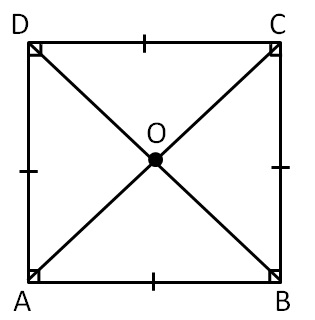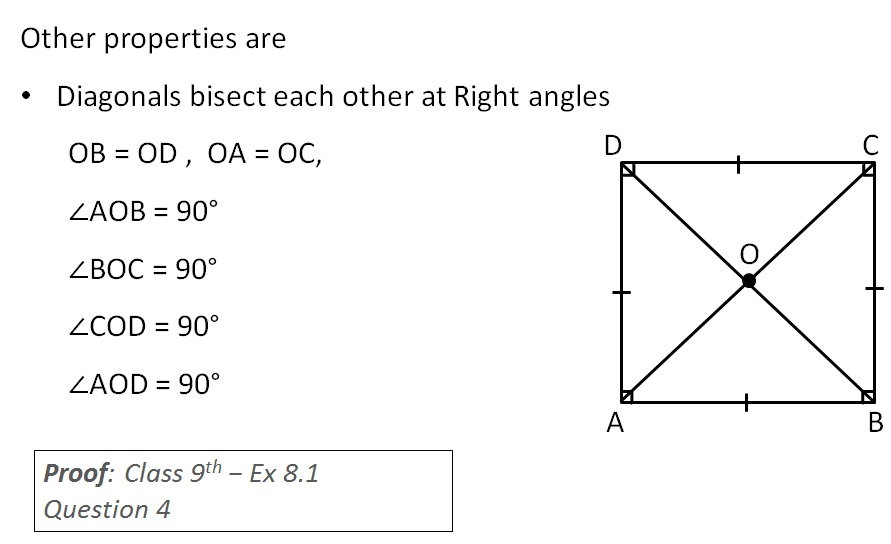Square is a parallelogram where

all sides are equal and all angles are 90°

Properties of Square:

Since it is a parallelogram,

it will have all its properties

In parallelogram

• Opposite sides are equal
• Opposite angles are equal
• Diagonals bisect each other
• Sum of adjacent angles is 180°

Other properties are

Diagonals are of equal length

i.e. AC = BD1. Chapter 4 Class 8 Practical Geometry
2. Concept wise
3. Constructing Square

Transcript

Other properties are Diagonals bisect each other at Right angles OB = OD , OA = OC, ∠AOB = 90° ∠BOC = 90° ∠COD = 90° ∠AOD = 90°

Constructing Square

About the AuthorDavneet Singh
Davneet Singh is a graduate from Indian Institute of Technology, Kanpur. He has been teaching from the past 9 years. He provides courses for Maths and Science at Teachoo.